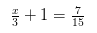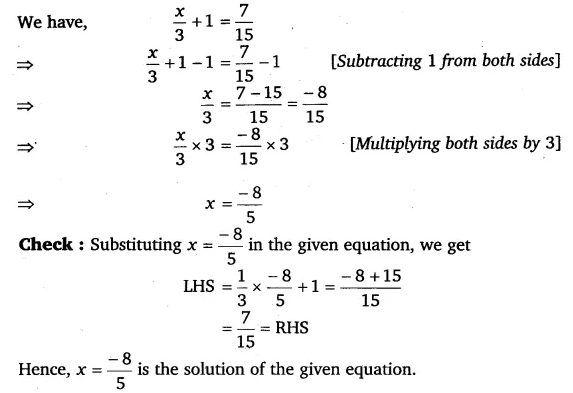# Solve the following equations: x/3 + 1 = 7/15

Solve the following equations: